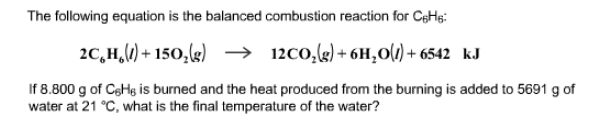# Problem: The following equation is the balanced combustion reaction for C6H6: 2C6H6(l) + 15O2(g) → 12CO2(g) + 6H2O(l) + 6542 kJIf 8.800 g of C6H6 is burned and the heat produced from the burning is added to 5691 g of water at 21 °C, what is the final temperature of the water?

###### FREE Expert Solution
87% (337 ratings)###### Problem Details

The following equation is the balanced combustion reaction for C6H6

2C6H6(l) + 15O2(g) → 12CO2(g) + 6H2O(l) + 6542 kJ

If 8.800 g of C6H6 is burned and the heat produced from the burning is added to 5691 g of water at 21 °C, what is the final temperature of the water?